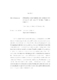## Twist-bulge derivatives and deformations of convex real projective structures on surfaces2015
##### Authors
Long, Terence Dyer
Let $S$ be a closed orientable surface with genus $g>1$ equipped with a convex $\mathbb{RP}^2$ structure. A basic example of such a convex $\mathbb{RP}^2$ structure on a surface $S$ is the one associated to a hyperbolic structure on $S$, and in this special case Wolpert proved formulas for computing the Lie derivatives $t_{\alpha}l_{\beta}$ and $t_{\gamma}t_{\alpha}l_{\beta}$, where $t_{\alpha}$ is the Fenchel-Nielsen twist vector field associated to the twist along a geodesic $\alpha$, and $l_{*}$ is the hyperbolic geodesic length function. In this dissertation, we extend Wolpert's calculation of $t_{\alpha}l_{\beta}$ and $t_{\gamma}t_{\alpha}l_{\beta}$ in the hyperbolic setting to the case of convex real projective surfaces; in particular, our $t_{\alpha}$ is the twist-bulge vector field along geodesic $\alpha$ coming from the parametrization of the deformation space of convex $\mathbb{RP}^2$ structures on a surface due to Goldman, and our geodesic length function $l_{*}$ is in terms of a generalized cross-ratio in the sense of Labourie. To this end, we use results due to Labourie and Fock-Goncharov on the existence of an equivariant flag curve associated to Hitchin representations, of which convex real projective surfaces are an example. This flag curve allows us to extend the notions arising in the hyperbolic case to that of convex real projective structures and to complete our generalization of Wolpert's formulas.